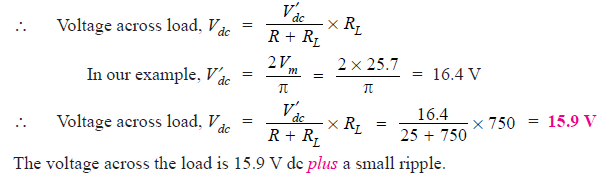# Solved Problems On Rectifiers

Q1. The applied input a.c. power to a half-wave rectifier is 100 watts. The d.c. output
power obtained is 40 watts.
(i) What is the rectification efficiency ?
(ii) What happens to remaining 60 watts ?

Solution :

(i)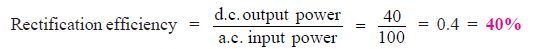(ii) 40% efficiency of rectification does not mean that 60% of power is lost in the rectifier circuit. In fact, a crystal diode consumes little power due to its small internal resistance. The 100 W a.c. power is contained as 50 watts in positive half-cycles and 50 watts in negative half-cycles. The 50 watts in the negative half-cycles are not supplied at all. Only 50 watts in the positive half-cycles are converted into 40 watts.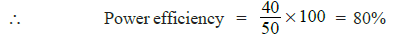Although 100 watts of a.c. power was supplied, the half-wave rectifier accepted only 50 watts and converted it into 40 watts d.c. power. Therefore, it is appropriate to say that efficiency of rectification is 40% and not 80% which is power efficiency.

Q2. An a.c. supply of 230 V is applied to a half-wave rectifier circuit through a transformer of turn ratio 10 : 1. Find (i) the output d.c. voltage and (ii) the peak inverse voltage. Assume the diode to be ideal.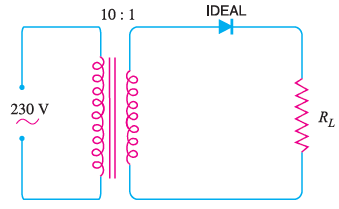Fig. 1

Solution :

Primary to secondary turns is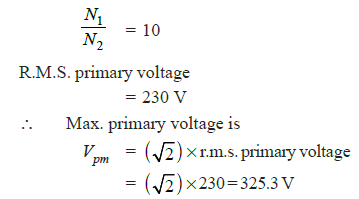Max. secondary voltage is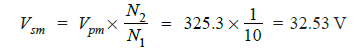(ii) During the negative half-cycle of a.c. supply, the diode is reverse biased and hence conducts no current. Therefore, the maximum secondary voltage appears across the diode.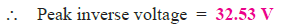Q3. A crystal diode having internal resistance r= 20Ω is used for half-wave rectification. If the applied voltage v = 50 sin ω t and load resistance RL= 800 Ω, find :
(i) Im, Idc, Irms (ii) a.c. power input and d.c. power output (iii) d.c. output voltage (iv) efficiency of rectification.

Solution :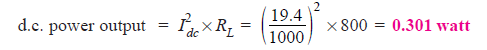Q4. A half-wave rectifier is used to supply 50V d.c. to a resistive load of 800 Ω. The diode has a resistance of 25 Ω. Calculate a.c. voltage required.

Solution :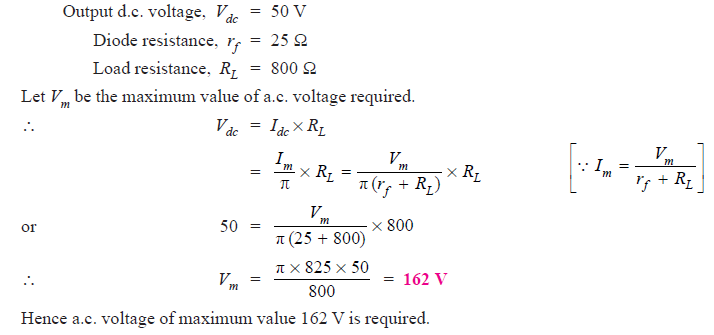Q5. A full-wave rectifier uses two diodes, the internal resistance of each diode may be assumed constant at 20 Ω. The transformer r.m.s. secondary voltage from centre tap to each end of secondary is 50 V and load resistance is 980 Ω. Find : (i) the mean load current (ii) the r.m.s. value of load current.

Solution :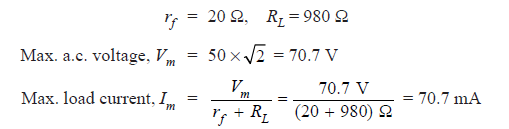Q6. In the centre-tap circuit shown in Fig. 2, the diodes are assumed to be ideal i.e. having zero internal resistance. Find :(i) d.c. output voltage(ii) peak inverse voltage (iii) rectification efficiency.Fig. 2

Solution :

Primary to secondary turns, N1 / N2 = 5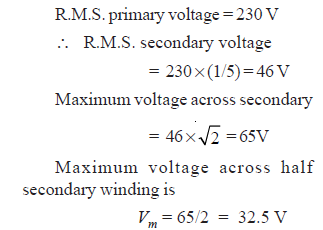(i) Average current, Idc =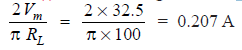(ii) The peak inverse voltage is equal to the maximum secondary voltage, i.e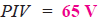(iii)Q7. In the bridge type circuit shown in Fig. 3, the diodes are assumed to be ideal. Find : (i) d.c. output voltage (ii) peak inverse voltage (iii) output frequency. Assume primary to secondary turns to be 4.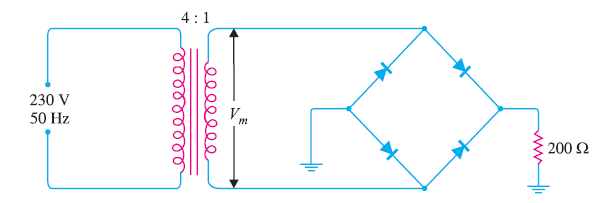Fig.3

Solution :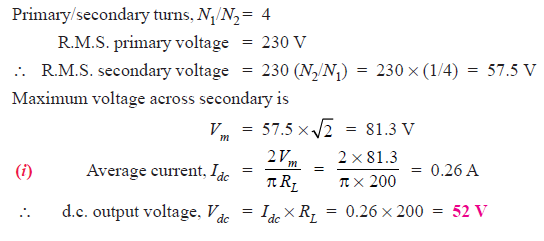Q8. Fig. 4(i) and Fig. 4 (ii) show the centre-tap and bridge type circuits having the same load resistance and transformer turn ratio. The primary of each is connected to 230V, 50 Hz supply. (i) Find the d.c. voltage in each case. (ii) PIV for each case for the same d.c. output. Assume the diodes to be ideal.

Solution :

(i) DC output voltage :

Centre-tap circuit :This shows that for the same secondary voltage, the d.c. output voltage of bridge circuit is twice that of the centre-tap circuit

(ii) PIV for same d.c. output voltage :

The d.c. output voltage of the two circuits will be the same if Vm (i.e. max. voltage utilized by each circuit for  conversion into d.c.) is the same. For this to happen, the turn ratio of the transformers should be as shown in Fig. 5.Fig.5

Centre-tap circuit :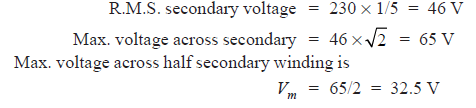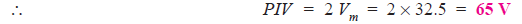Bridge circuit :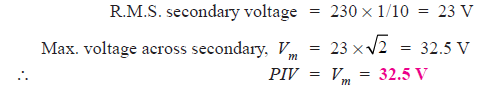This shows that for the same d.c. output voltage, PIV of bridge circuit is half that of centre-tap circuit. This is a distinct advantage of bridge circuit.

Q9. The four diodes used in a bridge rectifier circuit have forward resistances which may be considered constant at 1Ω and infinite reverse resistance. The alternating supply voltage is 240 V r.m.s. and load resistance is 480 Ω. Calculate (i) mean load current and (ii) power dissipated in each diode.

Solution :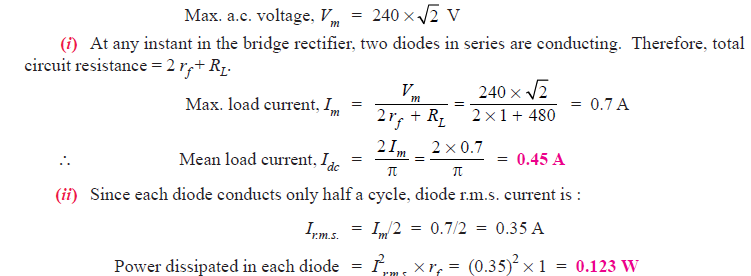Q10. The bridge rectifier shown in Fig. 6 uses silicon diodes. Find (i) d.c. output voltage (ii) d.c. output current. Use simplified model for the diodes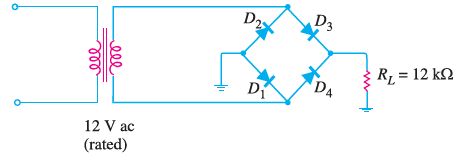Fig.6

Solution :

The conditions of the problem suggest that the a.c voltage across transformer secondary is 12V r.m.s.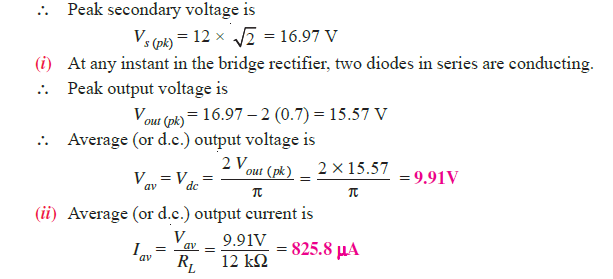Q11. A power supply A delivers 10 V dc with a ripple of 0.5 V r.m.s. while the power supply B delivers 25 V dc with a ripple of 1 mV r.m.s. Which is better power supply ?

Solution :

The lower the ripple factor of a power supply, the better it is.

For power supply A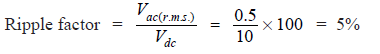For power supply B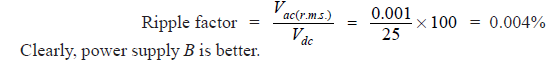Q12. For the circuit shown in Fig.7, find the output d.c. voltage.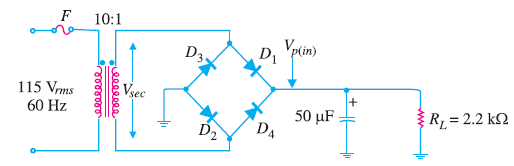Fig.7

Solution :

It can be proved that output d.c. voltage is given by :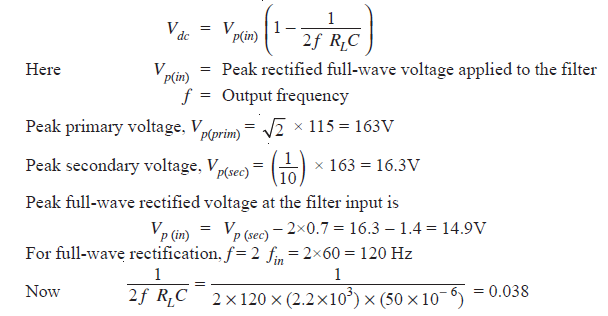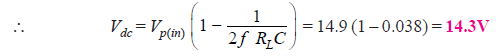Q13. The choke of Fig.8 has a d.c. resistance of 25 Ω. What is the d.c. voltage if the full-wave signal into the choke has a peak value of 25.7 V ?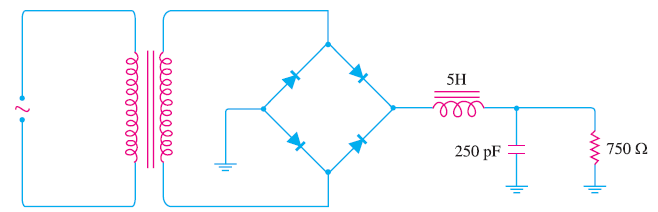Fig.8

Solution :

The output of a full-wave rectifier has a d.c. component and an a.c. component. Due to the presence of a.c. component, the rectifier output has a pulsating character as shown in Fig. 9.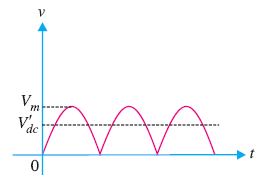Fig. 9

The maximum value of the pulsating output is Vm and d.c. component is V′dc = 2 Vm/π.

For d.c. component V′dc, the choke resistance is in series with the load as shown in 10.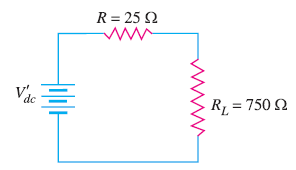Fig. 10